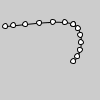# curve_point()#

Evaluates the curve at point t for points a, b, c, d.

## Examples#def setup():
py5.no_fill()
py5.curve(5, 26, 5, 26, 73, 24, 73, 61)
py5.curve(5, 26, 73, 24, 73, 61, 15, 65)
py5.fill(255)
py5.ellipse_mode(py5.CENTER)
steps = 6
for i in range(0, steps+1):
t = i / steps
x = py5.curve_point(5, 5, 73, 73, t)
y = py5.curve_point(26, 26, 24, 61, t)
py5.ellipse(x, y, 5, 5)
x = py5.curve_point(5, 73, 73, 15, t)
y = py5.curve_point(26, 24, 61, 65, t)
py5.ellipse(x, y, 5, 5)


## Description#

Evaluates the curve at point t for points a, b, c, d. The parameter t may range from 0 (the start of the curve) and 1 (the end of the curve). a and d are the control points, and b and c are points on the curve. As seen in the example, this can be used once with the x coordinates and a second time with the y coordinates to get the location of a curve at t.

Underlying Processing method: curvePoint

## Signatures#

curve_point(
a: float,  # coordinate of first control point
b: float,  # coordinate of first point on the curve
c: float,  # coordinate of second point on the curve
d: float,  # coordinate of second control point
t: float,  # value between 0 and 1
/,
) -> float


Updated on March 06, 2023 02:49:26am UTC